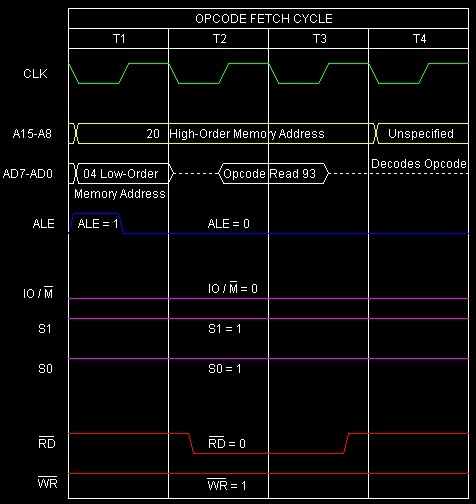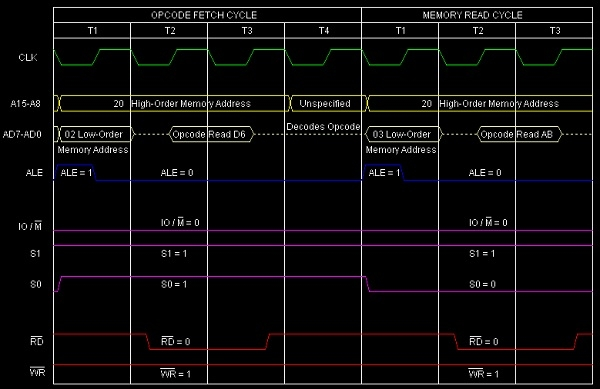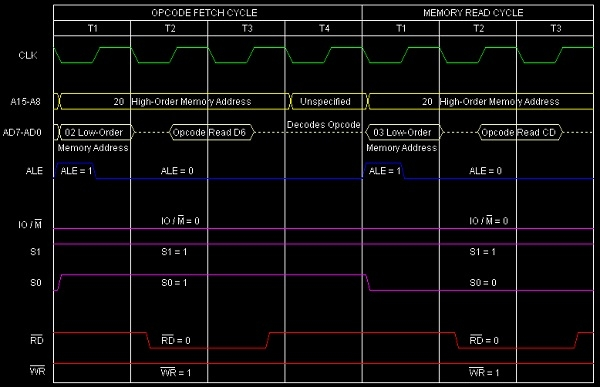# Instructions to perform subtraction in 8085 Microprocessor

In the subtraction of two numbers, 8085 imposes the restriction that Accumulator will have one of the operands from which the other operand specified by one of the following will be subtracted.

• —Contents of an 8-bit register;

• —Contents of memory location pointed by HL pair;

• —Eight-bit immediate data.

In 8085 Instruction, SUB is a mnemonic that stands for ‘SUBtract contents of R from Accumulator’. Here R stands for any of the following registers, or memory location M pointed by HL pair.

R = A, B, C, D, E, H, L, or M

Mnemonics, Operand
Opcode (in HEX)
Bytes
SUB A
97
1
SUB B
90
1
SUB C
91
1
SUB D
92
1
SUB E
93
1
SUB H
94
1
SUB L
95
1
SUB M
96
1

In this instruction content of the register R will get subtracted from the Accumulator and the resultant difference will be stored on the Accumulator replacing the previous content of the Accumulator. Where R can be any of the eight values, so as a result there are eight opcodes for this type of instruction as mentioned in the above table. It occupies only 1-Byte in the memory.

Let us consider SUB E as an example instruction of this category. As It is 1-Byte instruction so it will occupy a single Byte space in the memory. The result of execution of this instruction is shown below with examples.

Example 1:

Let us suppose Accumulator and E register are having initial values as ABH and CDH respectively. So after execution of the instruction SUB E the contents of the registers would be -

Before
After
(A)
ABH
DEH
(E)
CDH
CDH
(F)
Any values
Cy=1,AC=0,S=1,P=1,Z=0

Hex Codes
Mnemonic
Comment
2004
93
SUB E
Accumulator = Accumulator + 2’s Complement of E

Here Cy=1 indicates that result is negative. Actually A – E = A + 2’ Complement of E. Here is the calculation –

(A) BH (1010 1011) ---------------> 1010 1011

2'S Complement

(E) CDH (1100 1101) ---------------> 0011 0011

---------

1101 1110 (DEH)

Here is the timing diagram of the instruction SUB E as below -Summary: So this instruction SUB E requires 1-Byte, 1 Machine Cycles (Opcode Fetch) and 4 T-States for execution as shown in the timing diagram.

Example 2:

Let us suppose Accumulator and E register are having initial values as CDH and ABH respectively. So after execution of the instruction SUB E the contents of the registers would be -

Before
After
(A)
CDH
22H
(E)
ABH
ABH
(F)
Any values
Cy=0,AC=1,S=0,P=1,Z=0

Hex Codes
Mnemonic
Comment
2004
93
SUB E
Accumulator = Accumulator + 2’s Complement of E

Here Cy=0 indicates that result is positive. Actually A – E = A + 2’ Complement of E. Here is the calculation –

(A) CDH (1100 1101) ---------------> 1100 1101

2'S Complement

(E) ABH (1010 1011) ---------------> 0101 0101

---------

0010 0010 (22H)

Here is the timing diagram of the instruction SUB E as below -Summary: So this instruction SUB E requires 1-Byte, 1 Machine Cycles (Opcode Fetch) and 4 T-States for execution as shown in the timing diagram.

In 8085 Instruction set, SUI is a mnemonic that stands for ‘SUbtract Immediate from Accumulator’ and here d8 stands for any 8-bit or 1-Byte data. This instruction is used to subtract 8-bit immediate data from the Accumulator. The result of the subtraction will be stored in the Accumulator over witting its previous content. As it is an arithmetic instruction, so flag bits are affected based on the result. It is a 2-Byte instruction and occupies 2-Bytes in memory.

Mnemonics, Operand
Opcode (in HEX)
Bytes
SUI Data
D6
2

When we issue SUI d8 instruction then actually with the Accumulator’s content 2’s complement of d8 would get added. And the result will be stored back on the Accumulator.

Example 1:

SUI ABH is an example instruction of this type. It means from the Accumulator content, 2’s complement of ABH will get deducted. Let us consider the initial content of the Accumulator is CDH. Here is the tracing table –

Before
After
(A)
CDH
22H
(F)
Any values
Cy=0,AC=1,S=0,P=1,Z=0

Hex Codes
Mnemonic
Comment
2002
D6
SUI ABH
Accumulator = Accumulator + 2’s Complement of ABH
2003
AB

Operand ABH

Here Cy=0 indicates that result is positive. Actually A – ABH = A + 2’ Complement of ABH. Here is the calculation –

(A) CDH (1100 1101) ---------------> 1100 1101

2'S Complement

(d8) ABH (1010 1011) ---------------> 0101 0101

---------------

0010 0010 (22H)

Here is the timing diagram of the instruction SUI ABH instruction -Summary: So this instruction SUI d8 requires 2-Bytes, 2-Machine Cycles (Opcode Fetch, Memory Read) and 7 T-States for execution as shown in the timing diagram.

Example 1:

SUI ABH is an example instruction of this type. It means from the Accumulator content, 2’s complement of ABH will get deducted. Let us consider the initial content of the Accumulator is CDH. Here is the tracing table –

Before
After
(A)
CDH
22H
(F)
Any values
Cy=0,AC=1,S=0,P=1,Z=0

Hex Codes
Mnemonic
Comment
2002
D6
SUI ABH
Accumulator = Accumulator + 2’s Complement of ABH
2003
AB

Operand ABH

Here Cy=0 indicates that result is positive. Actually A – ABH = A + 2’ Complement of ABH. Here is the calculation –

(A) CDH (1100 1101) ---------------> 1100 1101

2'S Complement

(d8) ABH (1010 1011) ---------------> 0101 0101

--------------

0010 0010 (22H)

Here is the timing diagram of the instruction SUI ABH instruction -Summary: So this instruction SUI d8 requires 2-Bytes, 2-Machine Cycles (Opcode Fetch, Memory Read) and 7 T-States for execution as shown in the timing diagram.

Example 2:

SUI CDH is an example instruction of this type. It means from the Accumulator content, 2’s complement of CDH will get deducted. Let us consider the initial content of the Accumulator is ABH. Here is the tracing table –

 Before After (A) ABH DEH (F) Any values Cy=1,AC=0,S=1,P=1,Z=0

Hex Codes
Mnemonic
Comment
2002
D6
SUI CDH
Accumulator = Accumulator + 2’s Complement of CDH
2003
CD

Operand CDH

Here Cy=1 indicates that result is negative. Actually A – CDH = A + 2’ Complement of CDH. Here is the calculation –

ABH (1010 1011)       ---------------> 1010 1011

2'S Complement

(d8) CDH (1100 1101) ---------------> 0011 0011

--------------

1101 1110 (DEH)

Here is the timing diagram of the instruction SUI CDH instruction -Summary: So this instruction SUI d8 requires 2-Bytes, 2-Machine Cycles (Opcode Fetch, Memory Read) and 7 T-States for execution as shown in the timing diagram.